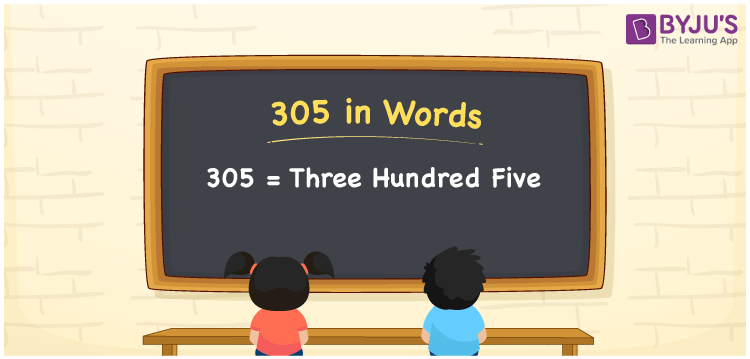# 305 in Words

Three hundred and five is written as 305 in words. In English, the number 305 is known as “Three Hundred Five.” Three hundred and fifty is a word that appears in a lot of real-life situations. As a result, it’s a counting number. We can also write Rupees Three Hundred Five or Three Hundred Five Rupees to signify the cash equal to 305. As a result, learning the numbers in words is crucial for understanding and expressing them.

 305 in words Three Hundred Five Three Hundred Five in Numbers 305

## 305 in English Words## How to Write 305 in Words?

If we know the place value of digits of 305, then we can easily express it in words. The place value is basically the position of a digit in a number. 305 is a three-digit number, therefore, we can specify the position of each digit of 305 in a place value chart. In the Indian numbering system, the order of place value of digits from right to left is given by:

 Hundreds Tens Ones 3 0 5

We can write it in expanded form as:

3 × Hundred + 0 × Ten + 5 × One

= 3 × 100 + 0 × 10 + 5 × 1

= 300 + 5

= 305

= Three Hundred Five

Therefore, 305 in words is written as eight hundred thirties.

305 is a natural number that precedes 306 and succeeds 304.

305 in words – Three Hundred Five

Is 305 an odd number? – Yes

Is 305 an even number? – No

Is 305 a perfect square number? – No

Is 305 a perfect cube number? – No

Is 305 a prime number? – No

Is 305 a composite number? – Yes

## Frequently Asked Questions on 305 in Words

Q1

### How to write 305 in words?

305 in English is written as “Three Hundred Five”.
Q2

### Is 305 an odd number or even number?

305 is an odd number because it is wholly not divisible by 2.
Q3

### Write Three Hundred Five in numbers.

Three Hundred Five in numbers is 305.